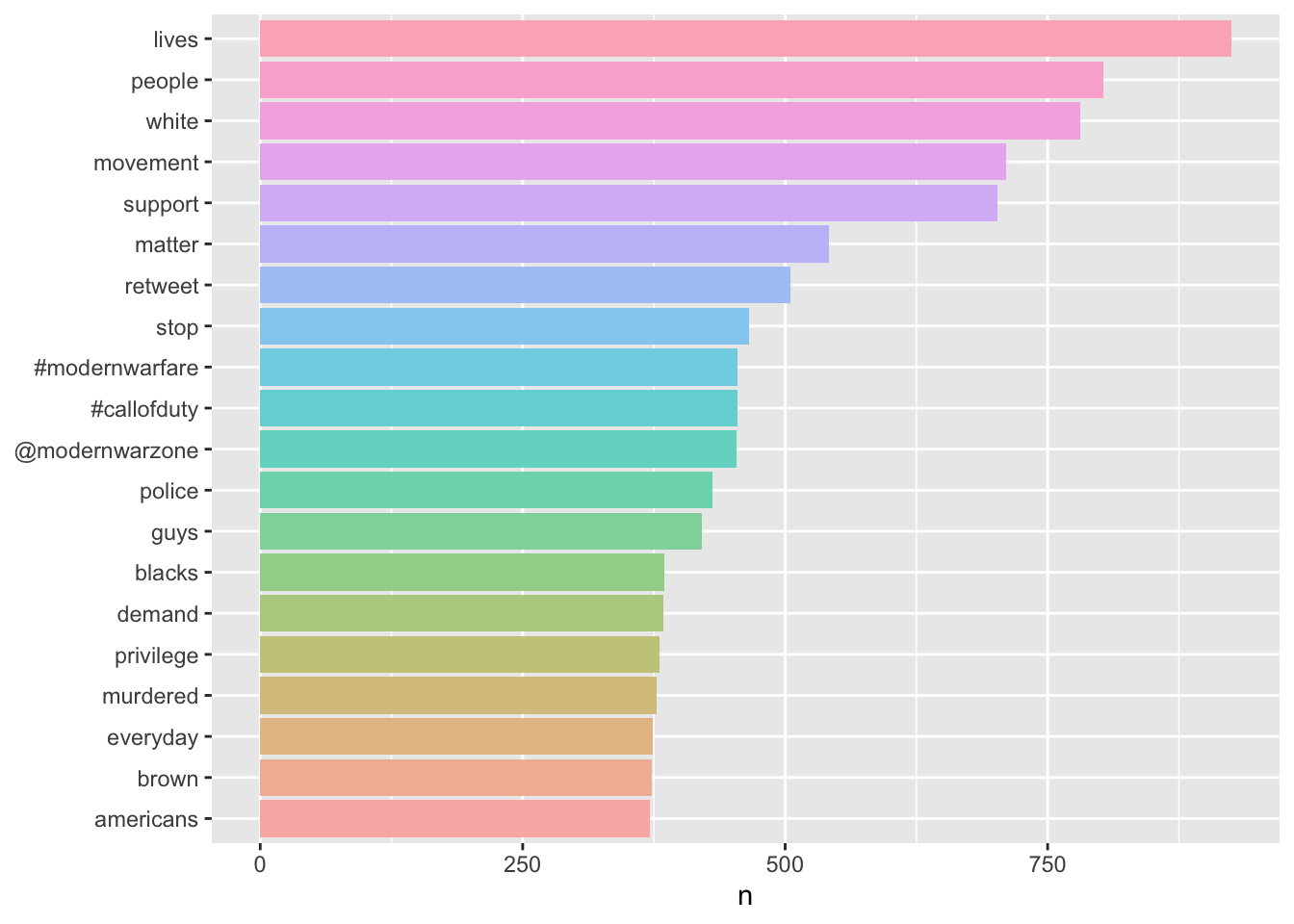# Background

This document is one of the Black Lives Matter projects that NYU Data Services staff members worked on in the Summer of 2020. The goal was to quantitatively analyze the reaction to George Floyd’s death and address its political consequences.

You’ll see how we use Twitter data to do sentiment analysis in R statistical programming language. The focus will be on text data wrangling, visualization, and interpretation with basic topic modeling at the end. You can download the code by clicking the “Code” button to the upper right.

First, we can install/load all of the packages that we will be using, and clear out the environment.

``````packages <- c("readxl","tidytext","plyr","dplyr","tidyr","ggplot2","scales",
"purrr","textdata","wordcloud","reshape2","stringr","igraph",
"ggraph","widyr","grid","arules","tm","topicmodels")
for(i in packages){
if(!require(i,character.only = T, quietly = T)){
install.packages(i)
}
library(i, character.only = T, quietly = T)
}

rm(list=ls())

#Set the seed to ensure that we get the same random numbers every time
#The seed could be any number you choose
set.seed(2020)``````

We will be using some twitter data web scrapped from June 2020 to August 2020. You can download the data set to your desktop by clicking here, save them in a folder called [data], then read them using the command below.

``````#Set working directory
setwd("~/Desktop")

#Read the data set for June

#Read the data set for July

#Read the data set for August

The `read_excel` function comes from the `readxl` package and is useful for reading excel data sets.

# Data Wrangling

## Data Clean Using Customized Functions

``````#create a list containing all six data frames
dfList<-list(tweets1,tweets2,tweets3,tweets4,tweets5,tweets6)

#create a function to do all the data clean process
result_list <- llply(dfList, function(x) {
#only keep tweets using English as the main language
x<-subset(x,x\$`19: Language`== "English")
#change the variable name for future convenience
x\$tweet=x\$`2: Tweet`
#drop all other variables except tweet
x<-x[,22]
#create a new variable to track the number of tweet
x\$tweetnumber<-1:length(x\$tweet)
#return the cleaner dataframe with 2 variables
return(x)
})

#apply the function to each dataset
twts1<-as.data.frame(result_list)
twts2<-as.data.frame(result_list)
twts3<-as.data.frame(result_list)
twts4<-as.data.frame(result_list)
twts5<-as.data.frame(result_list)
twts6<-as.data.frame(result_list)``````

The function gives us 6 data sets with only 2 variables: [tweet] and [tweetnumber]. We want to keep [tweetnumber] to track down every word from the tweet text later.

## Set Stop Words

``````#stop_words is a combination of English stop words from three lexicons, as a data frame.
data(stop_words)

#customize stop words
custom_stop_words <- bind_rows(
tibble(word = c("t.co","csun","blm","rt","https",
"BLM","blacklivesmatter","black",
"georgefloyd","2",'#blm','#blacklivesmatter',
'#georgefloyd','august'),
lexicon = c("custom")), stop_words)``````

We want to have some stop words for our analysis so that those wouldn’t appear in our frequency table and interfere our judgment.

## Extract Tokens

``````#store those special symbols in the variable so we can remove them later
remove_reg <- "&amp;|&lt;|&gt;"

#create a list containing all six data frames
dfList2<-list(twts1,twts2,twts3,twts4,twts5,twts6)
result_list2 <-
llply(dfList2, function(x) {
y <- x %>%
#remove special symbols for the values under the tweet variable
mutate(tweet = str_remove_all(tweet, remove_reg)) %>%
#extract every word from every tweet
unnest_tokens(word, tweet, token = "tweets") %>%
#filter out all stop words
filter(!word %in% custom_stop_words\$word,
!word %in%str_remove_all(custom_stop_words\$word, "'"),
str_detect(word, "[a-z]"))
return(y)})

tidy1<-as.data.frame(result_list2)
tidy2<-as.data.frame(result_list2)
tidy3<-as.data.frame(result_list2)
tidy4<-as.data.frame(result_list2)
tidy5<-as.data.frame(result_list2)
tidy6<-as.data.frame(result_list2)``````

Each single word in each tweet is considered as a token. By doing so, we can start to count frequency of each word or even each pair of words.

# Data Analysis and Visualization

## Visualize the Most Common Words

``````#Count the Frequency for Each Word
tidy_week11 <- tidy1 %>%dplyr::count(word, sort = TRUE)
tidy_week12 <- tidy2 %>%dplyr::count(word, sort = TRUE)
tidy_week21 <- tidy3 %>%dplyr::count(word, sort = TRUE)
tidy_week22 <- tidy4 %>%dplyr::count(word, sort = TRUE)
tidy_week31 <- tidy5 %>%dplyr::count(word, sort = TRUE)
tidy_week32 <- tidy6 %>%dplyr::count(word, sort = TRUE)

#Remove all non-english tokens
tidy1_english <- tidy_week11[which(!grepl("[^\x01-\x7F]+", tidy_week11\$word)),]
tidy2_english <- tidy_week12[which(!grepl("[^\x01-\x7F]+", tidy_week12\$word)),]
tidy3_english <- tidy_week21[which(!grepl("[^\x01-\x7F]+", tidy_week21\$word)),]
tidy4_english <- tidy_week22[which(!grepl("[^\x01-\x7F]+", tidy_week22\$word)),]
tidy5_english <- tidy_week31[which(!grepl("[^\x01-\x7F]+", tidy_week31\$word)),]
tidy6_english <- tidy_week32[which(!grepl("[^\x01-\x7F]+", tidy_week32\$word)),]``````
``````#create a list containing all six data frames
dfList3<-list(tidy1_english,tidy2_english,tidy3_english,tidy4_english,tidy5_english,tidy6_english)

#visualize using bar plot
result_list3 <-
llply(dfList3, function(x) {
plot <- x %>%
#keep only the top 20 tokens
dplyr::top_n(20) %>%
#reorder word based on the count
dplyr::mutate(word = reorder(word, n)) %>%
#plot using ggplot2
ggplot(aes(word, n, fill=word)) +
#specify it's a bar plot
geom_bar(stat="identity")+
scale_fill_hue(c=45, l=80)+
xlab(NULL) +
coord_flip()+
theme(legend.position="none")
return(plot)})

result_list3[]````````result_list3[]``